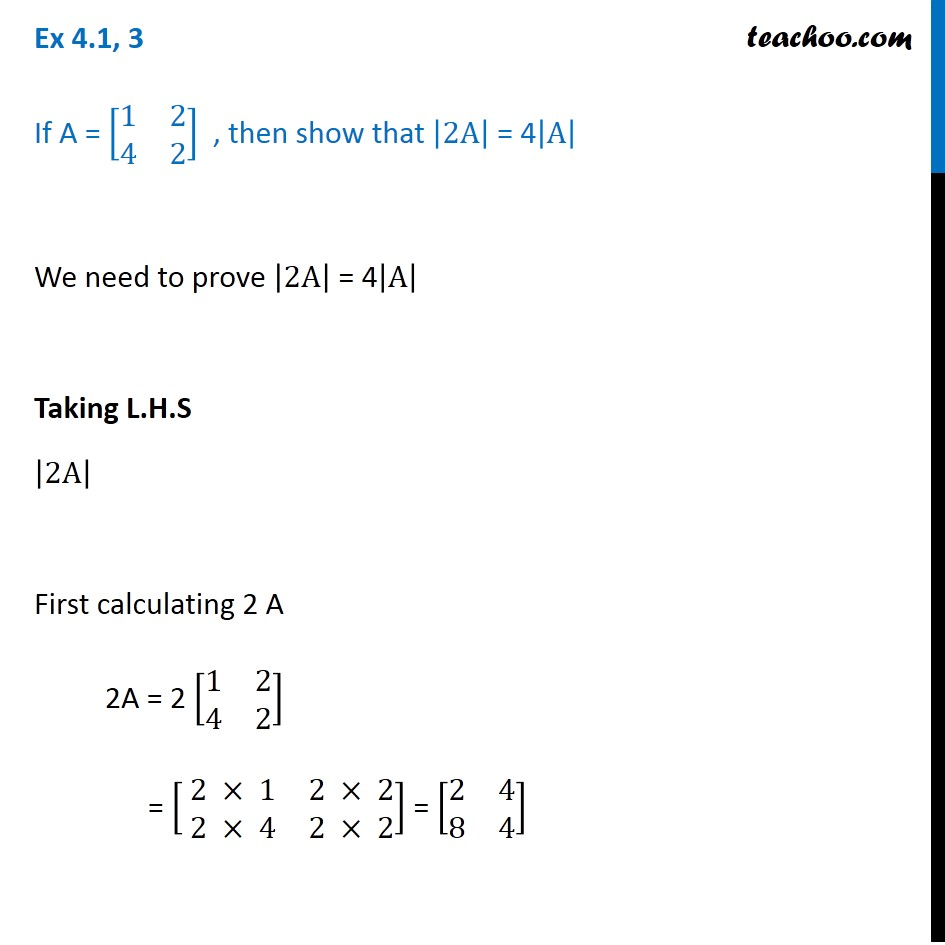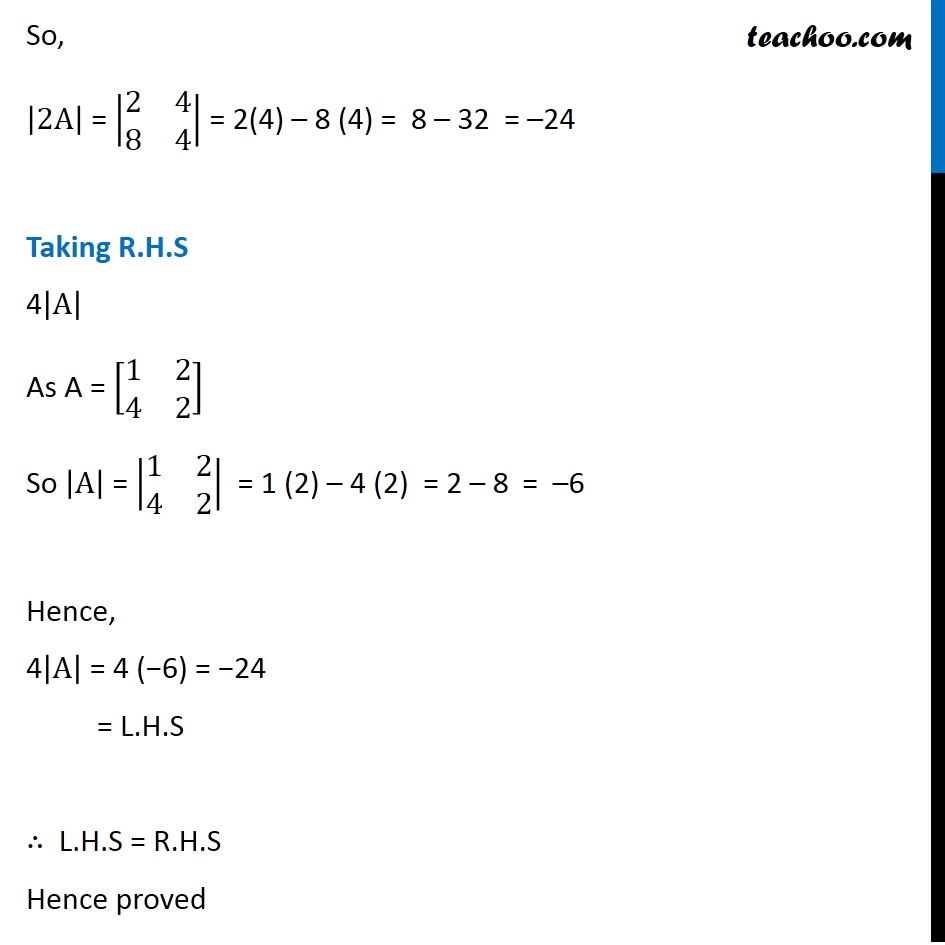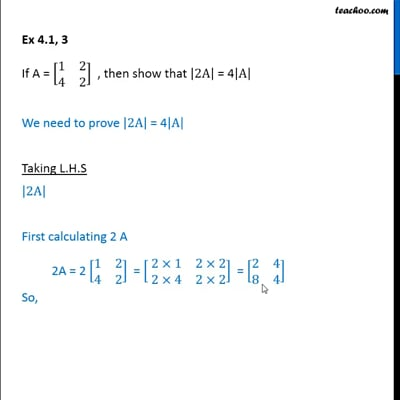Finding determinant of a 2x2 matrix

Chapter 4 Class 12 Determinants
Concept wiseThis video is only available for Teachoo black users

Introducing your new favourite teacher - Teachoo Black, at only ₹83 per month

### Transcript

Ex 4.1, 3 If A = [■8(1&2@4&2)] , then show that |2A| = 4|A| We need to prove |2A| = 4|A| Taking L.H.S |2A| First calculating 2 A 2A = 2 [■8(1&2@4&2)] = [■8( 2 × 1&2 × 2@ 2 × 4&2 × 2)] = [■8(2&4@8&4)] So, |2A| = |■8(2&4@8&4)| = 2(4) – 8 (4) = 8 – 32 = –24 Taking R.H.S 4|A| As A = [■8(1&2@4&2)] So |A| = |■8(1&2@4&2)| = 1 (2) – 4 (2) = 2 – 8 = –6 Hence, 4|A| = 4 (−6) = −24 = L.H.S ∴ L.H.S = R.H.S Hence proved